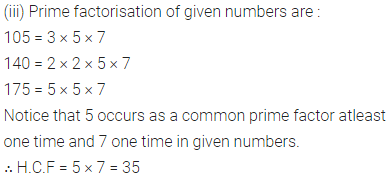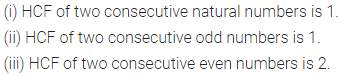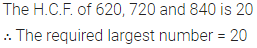# ML Aggarwal Class 6 Solutions for ICSE Maths Chapter 4 Playing with Numbers Ex 4.4

## ML Aggarwal Class 6 Solutions for ICSE Maths Chapter 4 Playing with Numbers Ex 4.4

Question 1.
Find the H.C.F. of the given numbers by prime factorisation method :
(i) 28, 36
(ii) 54, 72, 90
(iii) 105, 140, 175.
Solution:Question 2.
Find the H.C.F. of the given numbers by division method:
(ii) 198, 429
(ii) 20, 64, 104
(iii) 120, 144, 204
Solution:Question 3.
Fill in the blanks:
(i) HCF of two consecutive natural numbers is ……
(ii) HCF of two consecutive odd numbers is …..
(iii) HCF of two consecutive even numbers is ……
Solution:Question 4.
Find the greatest number which can divide 257 and 329 so as to leave a remainder 5 in each case.
Solution:Question 5.
Find the largest number that will divide 623, 729 and 841 leaving remainder 3, 9 and 1 respectively.
Solution:Question 6.
Meenu purchases two bags of rice of weights 75 kg and 69 kg. Find the maximum value of weight which can measure the weight of the rice exact number of times.
Solution:Question 7.
Three tankers contain 403 litres, 434 litres and 465 litres of diesel respectively. Find the maximum capacity of a container that can measure the diesel of three containers exact number of times.
Solution: